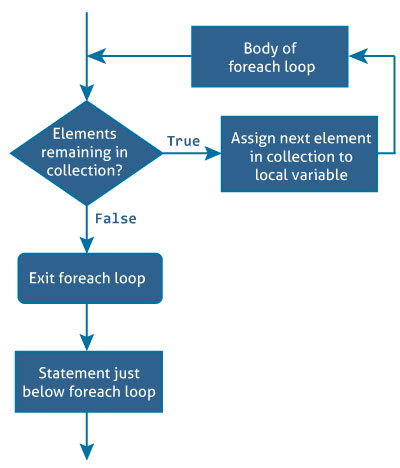C# foreach loop

# C# foreach loop

#### In this article, we will learn about foreach loops (an alternative to for loop) and how to use them with arrays and collections.

C# provides an easy to use and more readable alternative to for loop, the foreach loop when working with arrays and collections to iterate through the items of arrays/collections. The foreach loop iterates through each item, hence called foreach loop.

Before moving forward with foreach loop, visit:

## Syntax of foreach loop

```foreach (element in iterable-item)
{
// body of foreach loop
}
```

Here iterable-item can be an array or a class of collection.

## How foreach loop works?The `in` keyword used along with foreach loop is used to iterate over the iterable-item. The in keyword selects an item from the iterable-item on each iteration and store it in the variable element.

On first iteration, the first item of iterable-item is stored in element. On second iteration, the second element is selected and so on.

The number of times the foreach loop will execute is equal to the number of elements in the array or collection.

Here is an example of iterating through an array using the for loop:

## Example 1: Printing array using for loop

``````using System;

namespace Loop
{
class ForLoop
{
public static void Main(string[] args)
{
char[] myArray = {'H','e','l','l','o'};

for(int i = 0; i < myArray.Length; i++)
{
Console.WriteLine(myArray[i]);
}
}
}
}
``````

The same task can be done using the foreach loop.

## Example 2: Printing array using foreach loop

``````using System;

namespace Loop
{
class ForEachLoop
{
public static void Main(string[] args)
{
char[] myArray = {'H','e','l','l','o'};

foreach(char ch in myArray)
{
Console.WriteLine(ch);
}
}
}
}
``````

When we run the both program, the output will be:

```H
e
l
l
o
```

In the above program, the foreach loop iterates over the array, myArray. On first iteration, the first element i.e. myArray is selected and stored in ch.

Similarly on the last iteration, the last element i.e. myArray is selected. Inside the body of loop, the value of ch is printed.

When we look at both programs, the program that uses foreach loop is more readable and easy to understand. This is because of its simple and expressive syntax.

Hence, foreach loop is preferred over for loop when working with arrays and collections.

## Example 3: Traversing an array of gender using foreach loop

This program computes the number of male and female candidates.

``````using System;

namespace Loop
{
class ForEachLoop
{
public static void Main(string[] args)
{
char[] gender = {'m','f','m','m','m','f','f','m','m','f'};
int male = 0, female = 0;
foreach (char g in gender)
{
if (g == 'm')
male++;
else if (g =='f')
female++;
}
Console.WriteLine("Number of male = {0}", male);
Console.WriteLine("Number of female = {0}", female);
}
}
}
``````

When we run the program, the output will be:

```Number of male = 6
Number of female = 4
```

## Example 4: foreach loop with List (Collection)

This program computes the sum of elements in a List.

``````using System;
using System.Collections.Generic;

namespace Loop
{
class ForEachLoop
{
public static void Main(string[] args)
{
var numbers = new List() {5,-8,3,14,9,17,0,4};
int sum = 0;

foreach(int number in numbers)
{
sum += number;
}
Console.WriteLine("Sum = {0}", sum);
}
}
}
``````

When we run the program, the output will be:

`Sum = 44`

In this program, foreach loop is used to traverse through a collection. Traversing a collection is similar to traversing through an array.

The first element of collection is selected on the first iteration, second element on second iteration and so on till the last element.